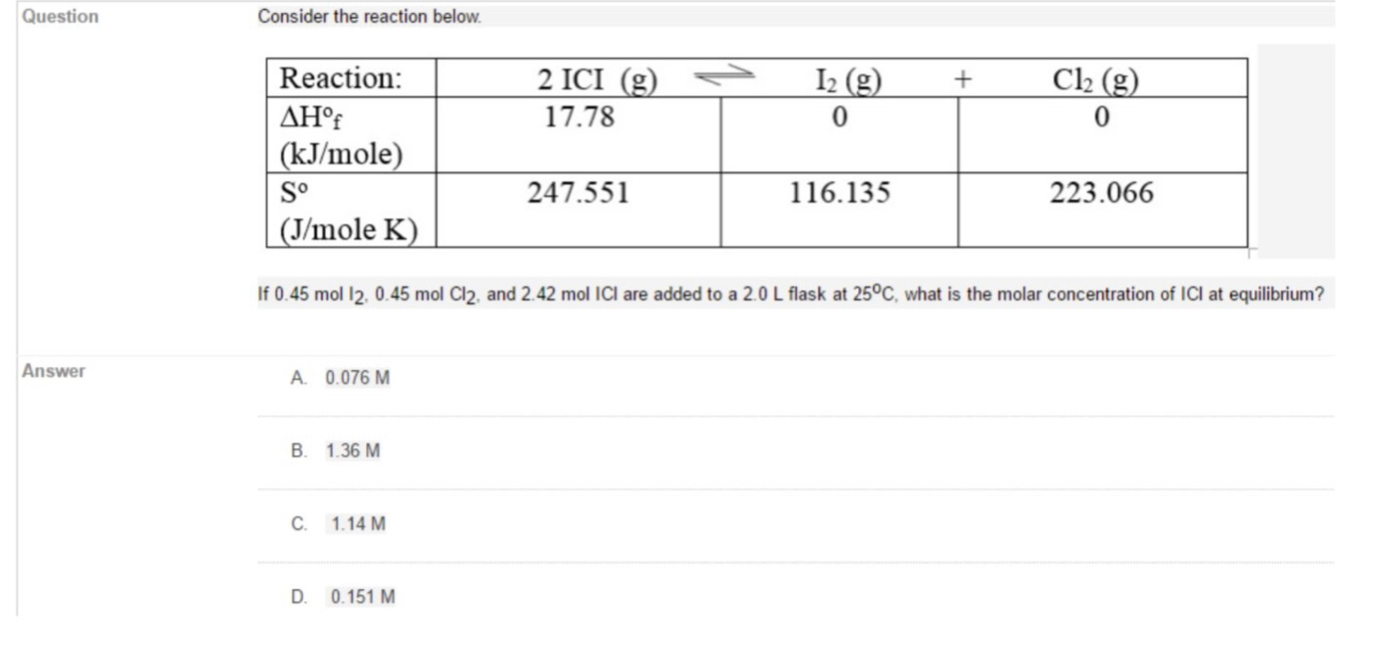# QuestionConsider the reaction below.|Reaction:C2 (g)I2 (g)2 ICI (g)<17.78AH°f| (kJ/mole)S°247.551116.135223.066(J/mole K)If 0.45 mol 12, 0.45 mol Cl2, and 2.42 mol ICI are added to a 2.0 L flask at 25°C, what is the molar concentration of ICI at equilibrium?AnswerA. 0.076 MB. 1.36 MC.1.14 MD. 0.151 M

Question
28 viewshelp_outlineImage TranscriptioncloseQuestion Consider the reaction below. |Reaction: C2 (g) I2 (g) 2 ICI (g) < 17.78 AH°f | (kJ/mole) S° 247.551 116.135 223.066 (J/mole K) If 0.45 mol 12, 0.45 mol Cl2, and 2.42 mol ICI are added to a 2.0 L flask at 25°C, what is the molar concentration of ICI at equilibrium? Answer A. 0.076 M B. 1.36 M C. 1.14 M D. 0.151 M fullscreen
check_circle

Step 1

The enthalpy change of the reaction is determined by subtracting enthalpy change of reactants from products as follows:

Step 2

The entropy change for the reaction is calculated by subtracting entropy of reactants from products as follows:

Step 3

The change in standard Gibbs free energy is related to enthalpy change and entropy change as giv...

### Want to see the full answer?

See Solution

#### Want to see this answer and more?

Solutions are written by subject experts who are available 24/7. Questions are typically answered within 1 hour.*

See Solution
*Response times may vary by subject and question.
Tagged in

### Equilibrium Concepts